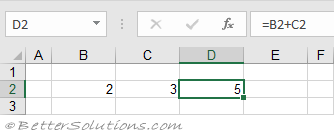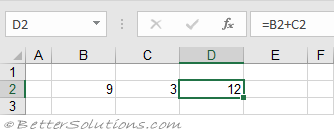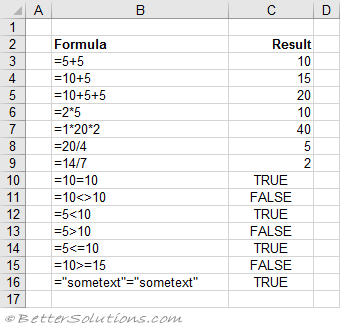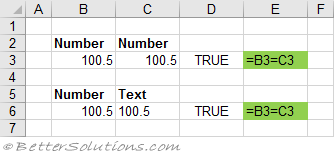# Inserting

Rather than entering your formulas through the Formula Bar you can just enter them directly into the cells.
This is the preferred method for a lot of people who are familiar with the function arguments.
Try to avoid hard coding values into formulas. Enter the data into cells and then reference the cells.
In this very simple example a formula is used in cell D2 to calculate the total of the values in cell B2 and C2.
The advantage of using a formula is that it is updated automatically, when the cells that it refers to change.
Once you have finished the formula, press Enter to confirm.
After you have pressed Enter the cell will display the result of the formula as opposed to the actual formula.If we change the value in cell B2 to 9, the formula is re-calculated automatically and the correct result is displayed.The table below shows the formula entered in column B and the result obtained in column C.All the formulas contain basic operations such as addition, subtraction, multiplication and division.
To represent these operations you use what are called Operators in between your numbers.
When you create a formula you need to include at least one operator.

### Equal To (=) Operator

A1 = B1
This will match even if one of the cells is formatted as a number and the other one is formatted as text